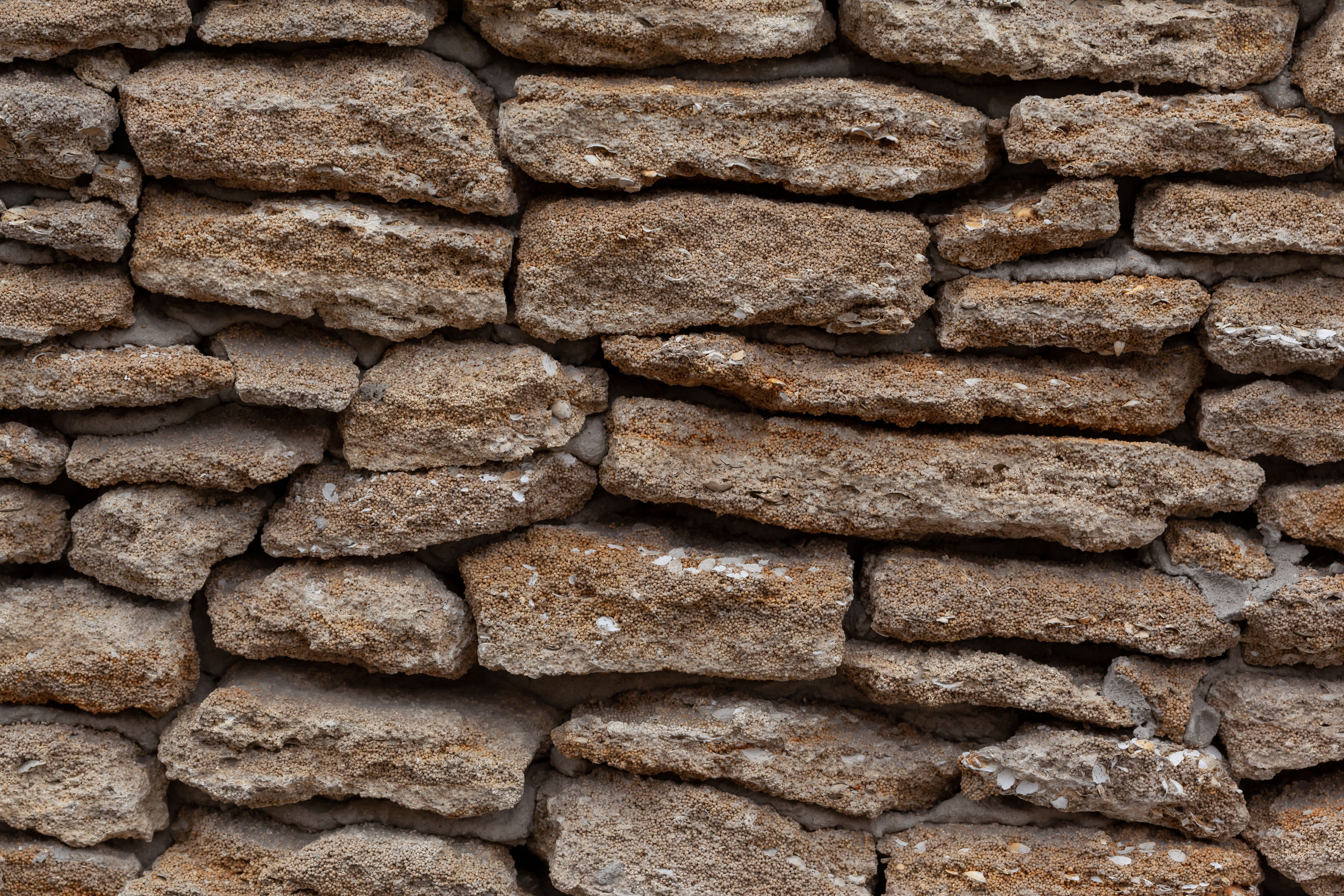# Is discrete math coding?

Is Discrete Math Coding?

# Is Discrete Math Coding?## Introduction

Discrete mathematics is a branch of mathematics that deals with discrete objects, such as integers, graphs, and statements in logic. It provides tools for reasoning about mathematical structures and functions, making it a foundation of computer science. In recent years, there has been ongoing debate about the role of coding in the teaching of discrete mathematics. In this article, we will explore whether discrete math is coding and how coding can be used to teach it.

## FAQs

### What Is Discrete Math?

Discrete mathematics is the study of mathematical structures that are countable or have a distinct or finite number of elements. This includes combinatorics, graph theory, set theory, and logic. Discrete mathematics is used in computer science, cryptography, and information theory, as well as in other areas of mathematics.Discrete math is essential for computer science because it provides the tools for reasoning about mathematical structures and functions, which are the building blocks of software systems. It enables computer scientists to design algorithms, analyze their performance, and optimize them for efficiency. Discrete math also plays a crucial role in cryptography, which is the science of secure communication, and in information theory, which is the study of the transmission of information.

Discrete mathematics is typically taught to computer science students as a foundation course in the first or second year of their degree. It is essential for mastering the programming concepts that follow in later years, such as data structures and algorithms.

### Is Discrete Math Coding?

While discrete math is not coding per se, it is closely related to computer science, and programming is often used to teach and apply its concepts. Discrete math provides the theoretical underpinnings for coding and computation, while coding turns these concepts into executable programs.The relationship between discrete math and coding can be seen in the use of discrete structures, such as graphs and trees, in algorithm design and analysis. Programmers use algorithms to solve problems in various domains, such as search engines, social media, and e-commerce. These algorithms rely on mathematical concepts such as set theory, Boolean logic, and probability.

In short, discrete math is a foundational concept that forms the basis of coding and computer science. While coding is not the same as discrete math, it is a practical application of its theoretical concepts and methods.

### How Can Coding Be Used to Teach Discrete Math?

Coding can be used to teach discrete math in several ways. Firstly, it provides a hands-on experience that helps students visualize and apply the concepts they learn in the classroom. Coding allows students to see how discrete structures work in practice and how algorithms can be applied to solve real-world problems.Secondly, coding can be used to provide immediate feedback to students. Most programming languages have built-in tools for debugging and testing code, which can help students find and correct errors in their programs. This feedback loop can be used to reinforce the concepts that students learn in class and help them develop problem-solving skills.